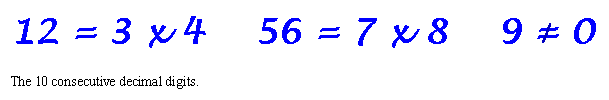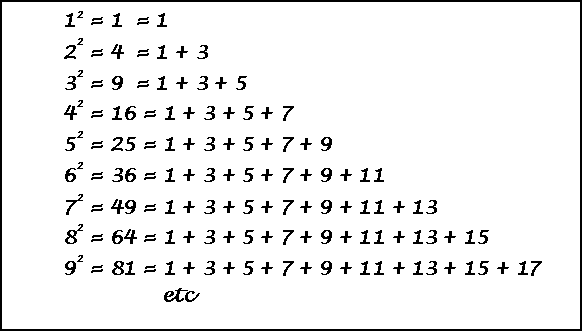# Miscellaneous Number PatternsThis is a small sampling from my collection of over 400 patterns. I hope you enjoy them.
And please call again soon.
Thanks to Dr. M. Ecker for adding the 9 <> 0 above.
See a picture of this pattern in cross-stitch, on my models page.

## Contents

### Introduction

A brief explanation of what this page is all about.

### Relation of consecutive odd numbers to squares.

The sum of N odd integers starting at 1 equals N squared.

### Relation of consecutive odd numbers to cubes

The sum of N odd integers starting after the previous series equals N cubed.

### Four Related Patterns

42, 342, 3342, etc. and three other similar numbers.

### 1089 and it's reversal

1089 x 9, 10989 x 9, etc., & their reversals x 4.

### Patterns with Primes

A page with 50+ prime number patterns.

### More Prime Patterns

Prime plots, Prime Queens problem, Prime rectangles, etc.

### Narcissistic Numbers

Patterns where the number equals some manipulation of its digits.

### Palindromes

A page of numbers that read the same backward as forward.

###Miscellaneous Patterns

New patterns added July 16, 2007## Introduction

Herein are presented a miscellany of number patterns and interesting numbers. They are displayed with very little commentary but with the hope they will increase the viewer's appreciation for the beauty that can be seen in mathematics.NOTE for elementary mathematics teachers; perhaps this material will be of value to your students for mathematics enrichment.

Recreational mathematics hobbyists. Can you add material or insight to these patterns?

See my policy on this web site regarding credits at All About Credits## Relation of consecutive odd numbers to squares.The sum of N odd integers starting at 1 equals N squared.## Relation of consecutive odd numbers to cubesThe sum of N odd integers starting after the previous series equals N cubed

### Four Related Patterns### 1089 and it's reversalThese are the only 4, 5, 6 and 7 digit numbers that are equal to a multiple of their reversal.
(The series may be extended indefinitely by inserting nines into the center of each number.)Miscellaneous Patterns

17 = 23 + 32     This is the only number of the form x + pq + qp .

17 = 34 – 43      Is this the only number of the form x + pq - qp ?

JRM 30:3, page 234. Problem 2447 proposed by Michael Ried.1729 = 103 + 93 = 123 + 13

Ramanujan observed that this is the smallest number with this property.
Ramanujan’s number plus the number of the beast equals the sum of the first prime and the squares of the next 9 primes.

1729 = 666 = 2 + 32 + 52 + 72 + 112 + 132 + 172 + 192 + 232 + 292 = 2395

Chanchal Singh, JRM 21:2, page 135. He also shows other facts about 1729.27 x 594 = 16038

This is the only example of the 10 digits appearing in numbers of 2, 3, and 5 digits in this form.
Also, 594 is also an exact multiple of 27.

The Canterbury Puzzles, H. E. Dudeney, Dover Publ., 1958, Puzzle 101, Page 2423816547290   is the only base 10 number that….. uses each of the 10 digits exactly once the leftmost k digits are evenly divisible by k. 3               is evenly divisible by 1 38               is evenly divisible by 2 381               is evenly divisible by 3 … 3816547290               is evenly divisible by 10. Mike Kieth, REC #91 (vol.13, No.5), Jan. 1999, page 11. 3608528850368400786036725 This is the largest integer that has the property that it’s leftmost k integers are evenly divisible by k (in this case 1 to 25). (Mike Kieth via email Jan. 20, 1999).(152 – 151 / 151) + 150 = 15 This works for any number. 2 x 9 = 18  and 92     =  81 1/243 = 004113226337448559124983/576 = 216.984375     digits 1 to 9 on each side of the equation. 2340819/576 = 4063.921875  digits 0 to 9 on each side of the equation.91 = 9
92 = 81
and 8 + 1 = 9
93 = 729
and 7 + 2 + 9 = 18             and 1 + 8 = 9
94 = 6561
and 6 + 5 + 6 + 1 = 18       and 1 + 8 = 9
95 = 59049
and 5 + 9 + 0 + 4 + 9 = 27 and 2 + 7 = 9

This pattern continues indefinitely .
This pattern looks good, but is the result of the simple fact that all multiples of 9 have a digital root of 9.

Matt Medlock (via email Nov. 21, 2002).(1 + 5 + 4 + 7)(12 + 52 + 42 + 72) = 1547
(2 + 1 + 9 + 6)(22 + 12 + 92 + 62) = 2196
Other numbers with this property are 1, 133, 315, 803, and 1148.

Aktar Yalcin (via email June 25, 2007).Last updated November 22, 2007
Harvey Heinz  harveyheinz@shaw.ca
Copyright © 1998 by Harvey D. Heinz